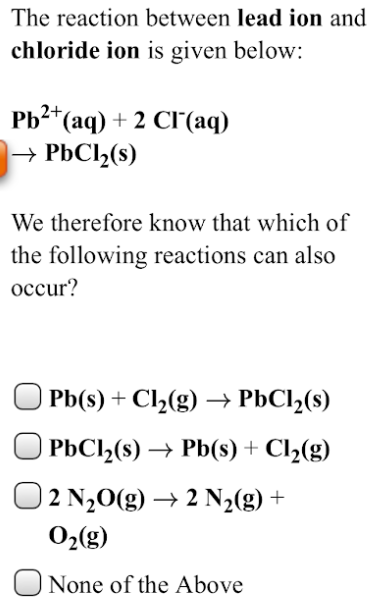# The reaction between lead ion and chloride ion is given below: Pb^2+(aq) + 2 Cl^-(aq) → PbCl2(s) We therefore know that which of the following reactions can also occur? a) Pb(s) + Cl2(g) → PbCl2(s) b) PbCl2(s) → Pb(s) + Cl2(g) c) 2 N2O(g) → 2 N2(g) + O2(g) d) None of the above